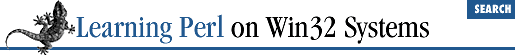home | O'Reilly's CD bookshelfs | FreeBSD | Linux | Cisco | Cisco Exam

### 4.3 The while/until Statement

No programming language would be complete without some form of iteration[ 2 ] (repeated execution of a block of statements). Perl can iterate using the ``` while``` statement:

 That's why HTML is not a programming language.

```while (```

some_expression
```
) {
```

statement_1
```
;
```

statement_2
```
;
```

statement_3
```
;
}```

To execute this ``` while``` statement, Perl evaluates the control expression (``` some_expression ``` in the example). If its value is true (using Perl's notion of truth), the body of the ``` while``` statement is evaluated once. This step is repeated until the control expression becomes false, at which point Perl goes on to the next statement after the ``` while``` ``` loop``` . For example:

```print "how old are you? ";
\$a = <STDIN>;
chomp(\$a);
while (\$a > 0) {
print "At one time, you were \$a years old.\n";
\$a--;
}```

Sometimes it is easier to say "until something is true" rather than "while not this is true." Once again, Perl has the answer. Replacing the ``` while``` with ``` until``` yields the desired effect:

```until (```

some_expression
```
) {
```

statement_1
```
;
```

statement_2
```
;
```

statement_3
```
;
}```

Note that in both the ``` while``` and ``` until``` forms, the body statements will be skipped entirely if the control expression is the termination value to begin with. For example, if a user enters an age less than zero for the program fragment above, Perl skips over the body of the loop.

Sometimes the control expression never lets the loop exit. This case is perfectly legal, and sometimes desired, and is thus not considered an error. For example, you might want a loop to repeat as long as you have no error, and then have some error handling code following the loop. You might use this for a program that is meant to run until the system terminates.4.2 The if/unless Statement4.4 The do {} while/until Statement